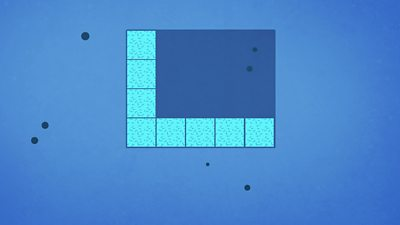# How can I work out the area of a shape?

## What is area?

Area means how much space a flat shape takes up.

Area is measured in square units:

• Square centimetres (cm²) are good for measuring a small area - like the picture or a model.
• Square metres (m²) are good for measuring bigger areas - like a wall or a playground.

## How do you work out the area of a shape?

You can work out the area of a shape by dividing it into squares and then counting all the squares. This can get a bit slow for big shapes.

### Is there a quicker way?

For squares and rectangles it can be quicker to measure the length, measure the breadth and then multiply them. So if a rectangle is 2 cm long and 3 cm wide, the area is:

2 cm x 3 cm = 6 cm²

## There's more to learn...

This guide